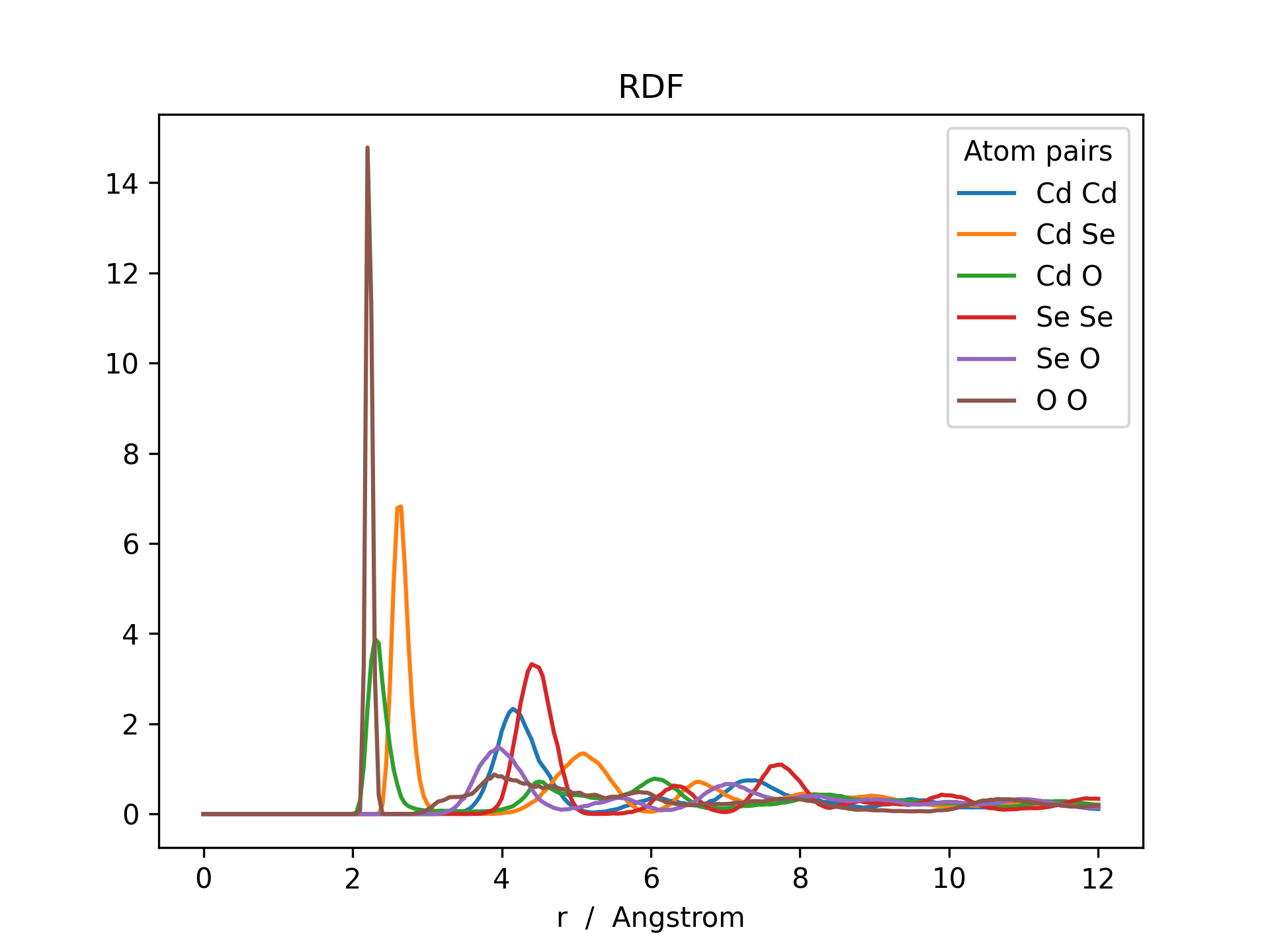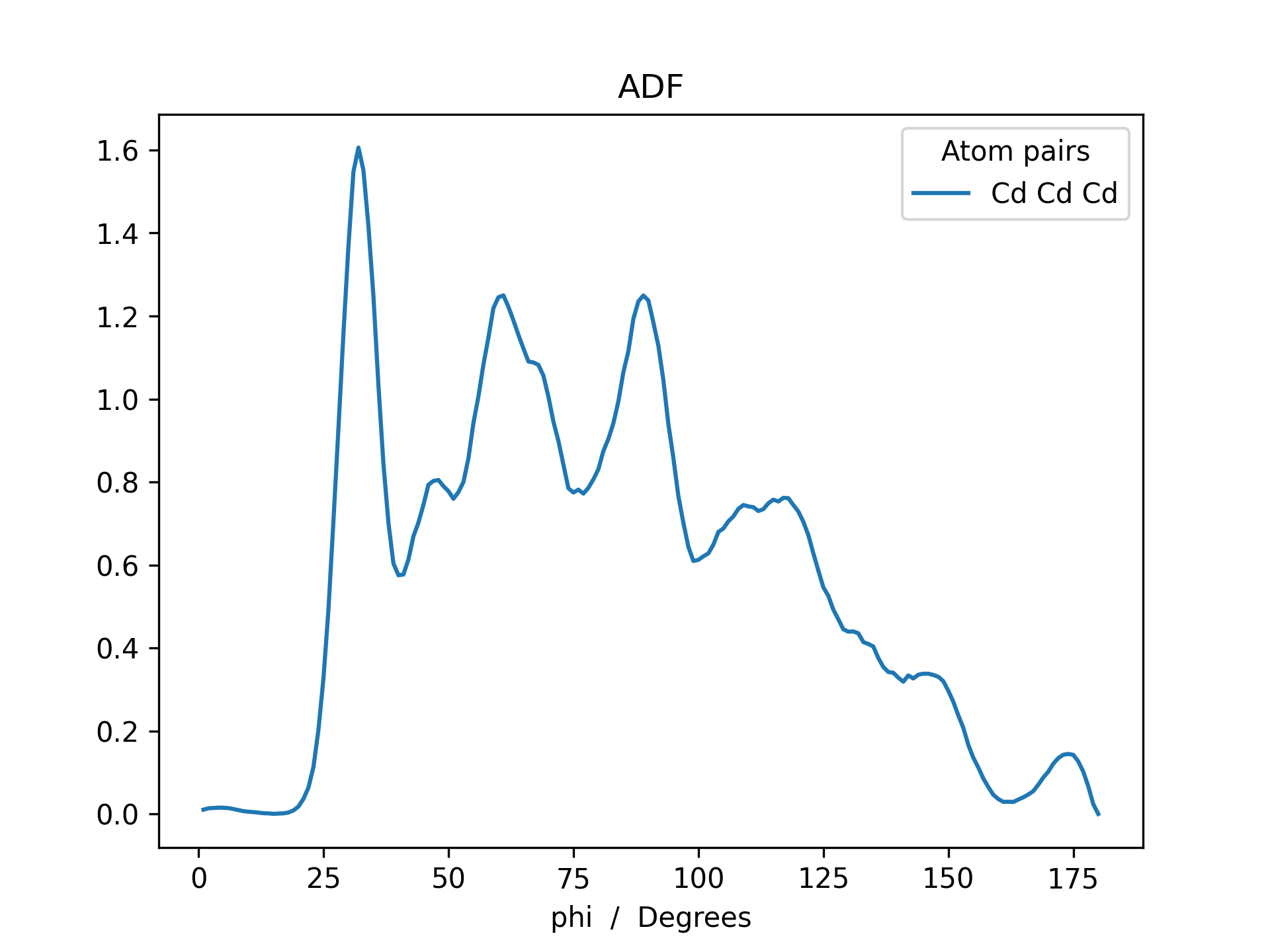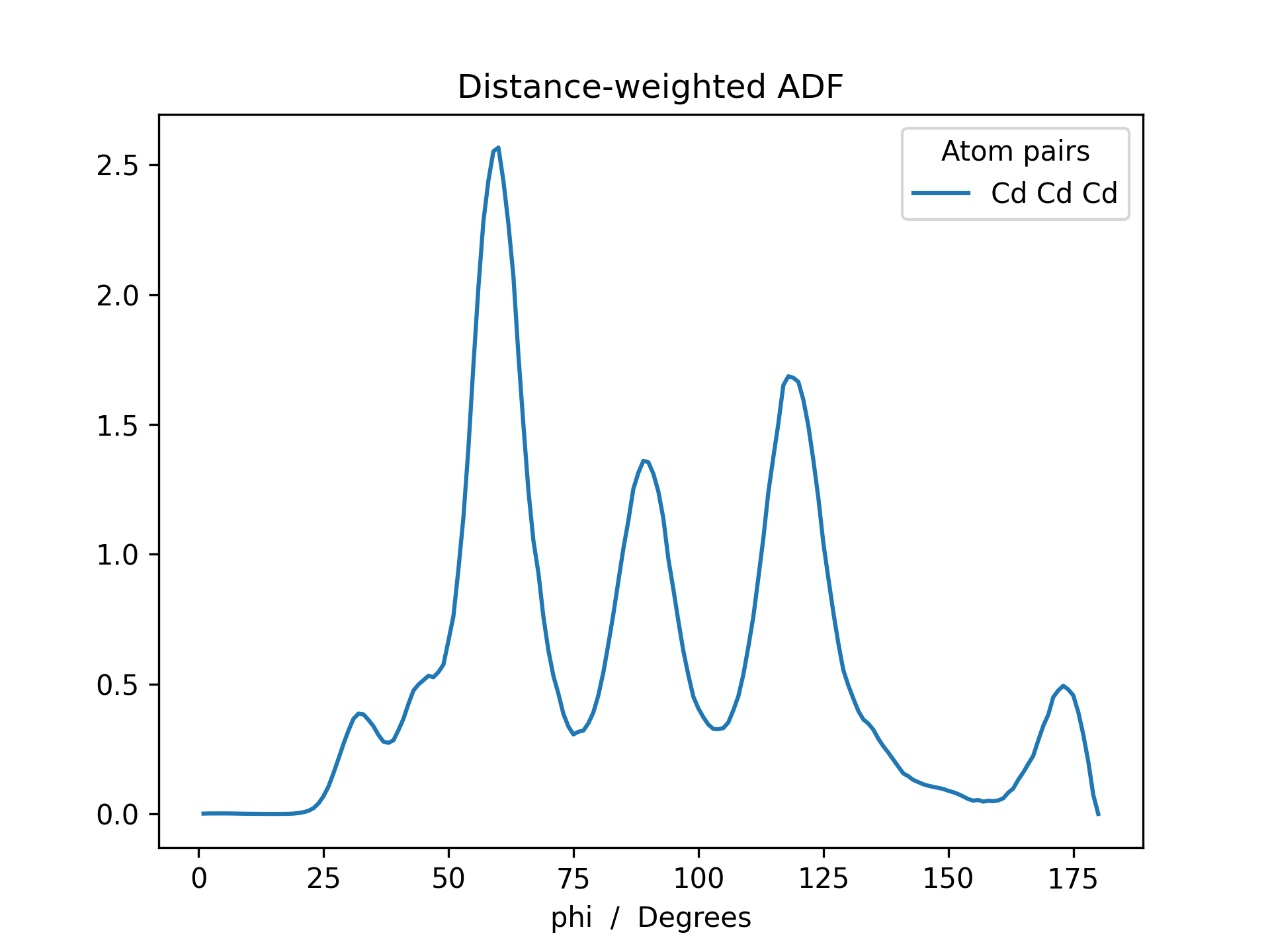# Radial & Angular Distribution Function¶

Radial and angular distribution function (RDF & ADF) generators have been implemented in the MultiMolecule class. The radial distribution function, or pair correlation function, describes how the particale density in a system varies as a function of distance from a reference particle. The herein implemented function is designed for constructing RDFs between all possible (user-defined) atom-pairs.

$g(r) = \frac{V}{N_a*N_b} \sum_{i=1}^{N_a} \sum_{j=1}^{N_b} \left< *placeholder* \right>$

Given a trajectory, mol, stored as a MultiMolecule instance, the RDF can be calculated with the following command: rdf = mol.init_rdf(atom_subset=None, low_mem=False). The resulting rdf is a Pandas dataframe, an object which is effectively a hybrid between a dictionary and a NumPy array.

A slower, but more memory efficient, method of RDF construction can be enabled with low_mem=True, causing the script to only store the distance matrix of a single molecule in memory at once. If low_mem=False, all distance matrices are stored in memory simultaneously, speeding up the calculation but also introducing an additional linear scaling of memory with respect to the number of molecules. Note: Due to larger size of angle matrices it is recommended to use low_mem=False when generating ADFs.

Below is an example RDF and ADF of a CdSe quantum dot pacified with formate ligands. The RDF is printed for all possible combinations of cadmium, selenium and oxygen (Cd_Cd, Cd_Se, Cd_O, Se_Se, Se_O and O_O).

>>> from FOX import MultiMolecule, example_xyz

>>> mol = MultiMolecule.from_xyz(example_xyz)

# Default weight: np.exp(-r)
>>> rdf = mol.init_rdf(atom_subset=('Cd', 'Se', 'O'))

>>> rdf.plot(title='RDF')## API¶

MultiMolecule.init_rdf(mol_subset=None, atom_subset=None, dr=0.05, r_max=12.0, mem_level=2)[source]

Initialize the calculation of radial distribution functions (RDFs).

RDFs are calculated for all possible atom-pairs in atom_subset and returned as a dataframe.

Parameters: mol_subset (slice) – Perform the calculation on a subset of molecules in this instance, as determined by their moleculair index. Include all $$m$$ molecules in this instance if None. atom_subset (Sequence) – Perform the calculation on a subset of atoms in this instance, as determined by their atomic index or atomic symbol. Include all $$n$$ atoms per molecule in this instance if None. dr (float) – The integration step-size in Ångström, i.e. the distance between concentric spheres. r_max (float) – The maximum to be evaluated interatomic distance in Ångström. mem_level (int) – Set the level of to-be consumed memory and, by extension, the execution speed. Given a molecule subset of size $$m$$, atom subsets of (up to) size $$n$$ and the resulting RDF with $$p$$ points (p = r_max / dr), the mem_level values can be interpreted as following: 0: Slow; memory scaling: $$n^2$$ 1: Medium; memory scaling: $$n^2 + m * p$$ 2: Fast; memory scaling: $$n^2 * m$$ A dataframe of radial distribution functions, averaged over all conformations in xyz_array. Keys are of the form: at_symbol1 + ‘ ‘ + at_symbol2 (e.g. "Cd Cd"). Radii are used as index. pd.DataFrame
MultiMolecule.init_adf(mol_subset=None, atom_subset=None, r_max=8.0, weight=<function neg_exp>)[source]

Initialize the calculation of distance-weighted angular distribution functions (ADFs).

ADFs are calculated for all possible atom-pairs in atom_subset and returned as a dataframe.

Parameters: mol_subset (slice) – Perform the calculation on a subset of molecules in this instance, as determined by their moleculair index. Include all $$m$$ molecules in this instance if None. atom_subset (Sequence) – Perform the calculation on a subset of atoms in this instance, as determined by their atomic index or atomic symbol. Include all $$n$$ atoms per molecule in this instance if None. r_max (float or str) – The maximum inter-atomic distance (in Angstrom) for which angles are constructed. The distance cuttoff can be disabled by settings this value to np.inf, "np.inf" or "inf". weight (Callable[[np.ndarray], np.ndarray], optional) – A callable for creating a weighting factor from inter-atomic distances. The callable should take an array as input and return an array. Given an angle $$\phi_{ijk}$$, to the distance $$r_{ijk}$$ is defined as $$max[r_{ij}, r_{jk}]$$. Set to None to disable distance weighting. A dataframe of angular distribution functions, averaged over all conformations in this instance. pd.DataFrame

Note

Disabling the distance cuttoff is strongly recommended (i.e. it is faster) for large values of r_max. As a rough guideline, r_max="inf" is roughly as fast as r_max=15.0 (though this is, of course, system dependant).

Note

The ADF construction will be conducted in parralel if the DASK package is installed. DASK can be installed, via anaconda, with the following command: conda install -n FOX -y -c conda-forge dask.